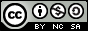- 目录 -
LeetCode 983 最低票价(dp)

• 一张为期一天的通行证售价为 costs 美元；
• 一张为期七天的通行证售价为 costs 美元；
• 一张为期三十天的通行证售价为 costs 美元。

输入：days = [1,4,6,7,8,20], costs = [2,7,15]



输入：days = [1,2,3,4,5,6,7,8,9,10,30,31], costs = [2,7,15]



1. 1 <= days.length <= 365
2. 1 <= days[i] <= 365
3. days 按顺序严格递增
4. costs.length == 3
5. 1 <= costs[i] <= 1000

## 思路

1. 买第i天当天的票，花费costs元，即 dp[i]=dp[i-1]+costs
2. 7天前买的票正好延续到今天，花费即为：dp[i]=dp[i-7]+costs
3. 30天前买的票正好延续到今天，花费为：dp[i]=dp[i-30]+costs

## 代码

  1 2 3 4 5 6 7 8 9 10 11 12 13 14 15 16 17 18 19 20  class Solution { public: int mincostTickets(vector &days, vector &costs) { unordered_map mp; for (int day : days) mp[day]++; int n = days.size(); vector dp(days[n - 1] + 1, 0); for (int i = 1; i <= days[n - 1]; i++) { if (mp[i]) dp[i] = min(min(dp[i - 1] + costs, dp[i - 7 > 0 ? i - 7 : 0] + costs), dp[i - 30 > 0 ? i - 30 : 0] + costs); else dp[i] = dp[i - 1]; } return dp[days[n - 1]]; } };